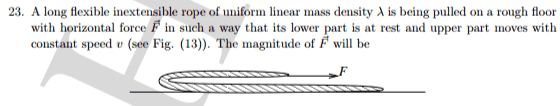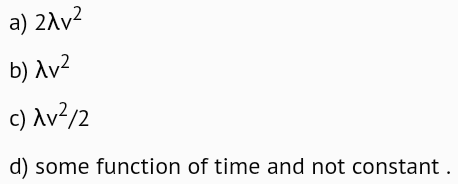# Force required to pull a rope

## Homework Statement## The Attempt at a Solution

Suppose length of upper portion is ##x## and that of lower portion is ##L-x## .

In time ##dt## , ##dm## mass of rope starts moving abruptly with speed ##v## .

The kinetic friction acting on the upper part of the rope is ##f = \mu M(x)g## , where M(x) = Mx/L .

dx = Mdx/L

Applying impulse momentum theorem ,

##(F-\mu \lambda xg)dt = (m+dm)(v) - mv##

##(F-\mu \lambda xg)dt = vdm##

I don't know how to proceed from here .

I would appreciate if someone could guide me .

Thanks

#### Attachments

Last edited:

kuruman
Homework Helper
Gold Member
Forget kinetic friction. You know that
$$F=\frac{dp}{dt}=M\frac{dv}{dt}+v\frac{dM}{dt}$$
Find ##dv/dt##.
Find an expression for ##M## (moving mass) as a function of time then take the derivative.
Substitute.

Forget kinetic friction.

Why ?

If my system is the entire rope , the external forces are kinetic friction and the pulling force F .

Please point out mistake in the OP .

kuruman
Homework Helper
Gold Member
Why ?
Because you don't need it. When length ##dx## is added to the rope, the pulling force does just enough work to bring additional mass ##\lambda~dx## to final speed ##v##.

You asked for guidance and I gave it to you. Just follow the three steps that I outlined and you will see what's actually going on in relation to what you initially thought.

The system is only the part of the rope that is moving and has variable mass. For all you care, you may have an infinite rope lying on the table and the answer will not be different.

Please confirm one thing .Is the upper moving part of the rope sliding on the surface or is it in air ?

kuruman
Homework Helper
Gold Member
The picture you posted shows that it is in air.

The picture you posted shows that it is in air.

Alright . Thanks . I was assuming upper part to be sliding on the rough surface .

If the upper part of rope is in air , then from the OP , I can put friction f=0 and F = vdm/dt = λv2 , i.e option b) ?

kuruman
Homework Helper
Gold Member
Yes.

Yes.

According to answer key correct option is c)I saw the same question in another book . It also suggests answer is option c) .

Last edited:
Orodruin
Staff Emeritus
Homework Helper
Gold Member
Clearly you cannot support the rope in the air like that, but I do believe you are supposed to neglect kinetic friction.

Option c is only correct if the work-energy theorem applies rather than momentum conservation, but I would say this is a typical case where it would not (essentially you have inelastic collision of the moving rope and the part that starts to move). I would go with b.

haruspex
Homework Helper
Gold Member
2020 Award
Option c is only correct if the work-energy theorem applies rather than momentum conservation, but I would say this is a typical case where it would not (essentially you have inelastic collision of the moving rope and the part that starts to move). I would go with b.
I got option c using momentum. Certainly work conservation should not be assumed.
In time t, the leading end of the rope advaces by vt and the fold advances by vt/2. This means that a length of rope vt/2, previously stationary, is now moving at speed v. All other sections of rope are at unchanged velocities. The change in momentum is λv2t/2.

•Vibhor
Orodruin
Staff Emeritus
Homework Helper
Gold Member
You are right. Forgot about the motion of the fold (as did OP I suspect).

(b) would be correct only if the rope was lying in a (very idealised) pile.

•Vibhor
In time t, the leading end of the rope advaces by vt and the fold advances by vt/2.

How did you infer the distance moved by the fold ?

I understand that when the leading end moves by vt , vt/2 amount of rope from top and vt/2 from bottom starts moving , but essentially the mass that has moved is λvt .

Orodruin
Staff Emeritus
Homework Helper
Gold Member
but essentially the mass that has moved is λvt .
No, it is not. When ##vt## is equal to the length of the rope, only half the rope has started moving - the other half is still at rest.

The top part of the rope is already moving.

•Vibhor
No, it is not. When ##vt## is equal to the length of the rope, only half the rope has started moving - the other half is still at rest.

If top end where force is applied moves a distance vt , why shouldn't vt length of the rope be moving ? Isn't distance moved by top end be same as the length of additional part of the rope that has started moving ?

Sorry for being slow in understanding this . I might be having difficulty in relating speed of the moving end and the length of rope that acquires speed .

haruspex
Homework Helper
Gold Member
2020 Award
Isn't distance moved by top end be same as the length of additional part of the rope that has started moving ?
If at time 0 we label the point on the rope at the fold as A, at time t A has moved vt to the right. But the new fold, as a point on the table, is vt/2 from where the old fold was, so the extra length in motion is from the new fold to A, a distance vt/2.

•Vibhor

When rope end moves from F to F' by distance z , fold moves from A to A' by distance z/2 . OK till here .

But in the process doesn't part CD (from top) and part EG (from bottom) move by distance z/2 each .In effect z length of rope movesLast edited:
.

Last edited:
haruspex
Homework Helper
Gold Member
2020 Award
doesn't part CD (from top) and part EG (from bottom) move by distance z/2 each
Yes, but CD was already in motion and has not changed velocity. All the momentum change is in EG.

•Vibhor
Yes, but CD was already in motion and has not changed velocity. All the momentum change is in EG.

RightThanks a lot !

Thank you very much @Orodruin .